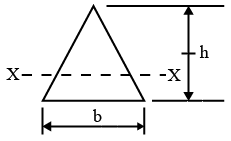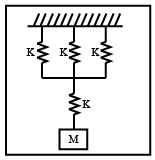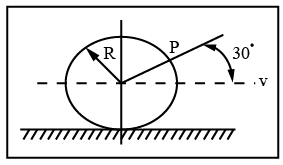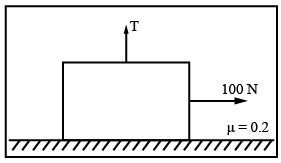# ISRO Scientist or Engineer Mechanical 2012

Instructions

For the following questions answer them individually

Question 1

# A beam is having-a-triangular cross section with its neutral axis XX. The section modulus about XX is given byQuestion 2

# A rectangular column is subjected to an eccentric load P at distance ‘e’ from centroidal Axis. The stress diagram at Cross- section will beQuestion 3

# Two shafts A & B are made of same material. The radius of the shaft A is half that of shaft B. The power transmitted by the shaft A will be ___________ that of shaft B.Question 4

# Four linear elastic springs are connected to mass ‘M’ as shown in Figure. The natural frequency of the system isQuestion 5

# Which bearing offers lowest frictionQuestion 6

# A circular disk of radius R rolls without slipping at a velocity V. The magnitude of the velocity at point P shown in the figure isQuestion 7

# In the viscous damped vibration, the logarithmic decrement value over five cycles is found to be 8.11. What is viscous damping factor of vibratory system?Question 8

# An elevator weighing 1000Kg attains an upward velocity of 4m/sec in two seconds with uniform acceleration. The tension (in N) in supporting cable will be $$(g = 9.8m/sec^2)Question 9 # A block weighting 981 N is resting on Horizontal surface. The co-efficient of friction between the block and horizontal surface is$$\mu = 0.2. A vertical cable attached to block provides partial support as shown. A man can pull horizontally with a force of 100 N. What will be the tension (T)(in N)in the cable if the man is just able to move the block to the right?Question 10

# A vehicle suspension system consists of a leaf spring and a damper. The stiffness of the leaf spring is 3.6 KN/m and damping constant of the damper is 400 Ns/m. If the mass is 50Kg, then the damping factor and damped natural frequency respectively areOR